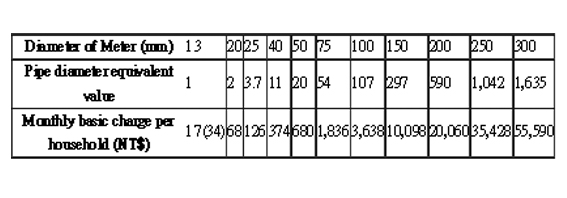﻿ Taipei Water Department-FAQ-What is the Basis for calculation of basic charges ?
Jump to the content zone at the center

### What is the Basis for calculation of basic charges ?

Based on (1) the standard basic charge of NT\$34 monthly per household installed with a 13 mm water meter and (2) the pipe diameter equivalent value of all other sized meters, basic charges for all households are acquired by multiplying NT\$34 by the equivalent values. Low-income households installed with 13 mm meters pay half of the standard basic charge, or NT\$17.To exemplify, let’s compare a13 mm and a 100 mm meters. Assuming same water pressure and friction coefficient, then the volume of water flow from a single 100 mm pipe equals the combined volume of water flows from 107 pieces of 13 mm pipes. Therefore, the basic charge of a household using a 100 mm meter = that using a 13 mm meter (NT\$34) x 107 = NT\$3,638.